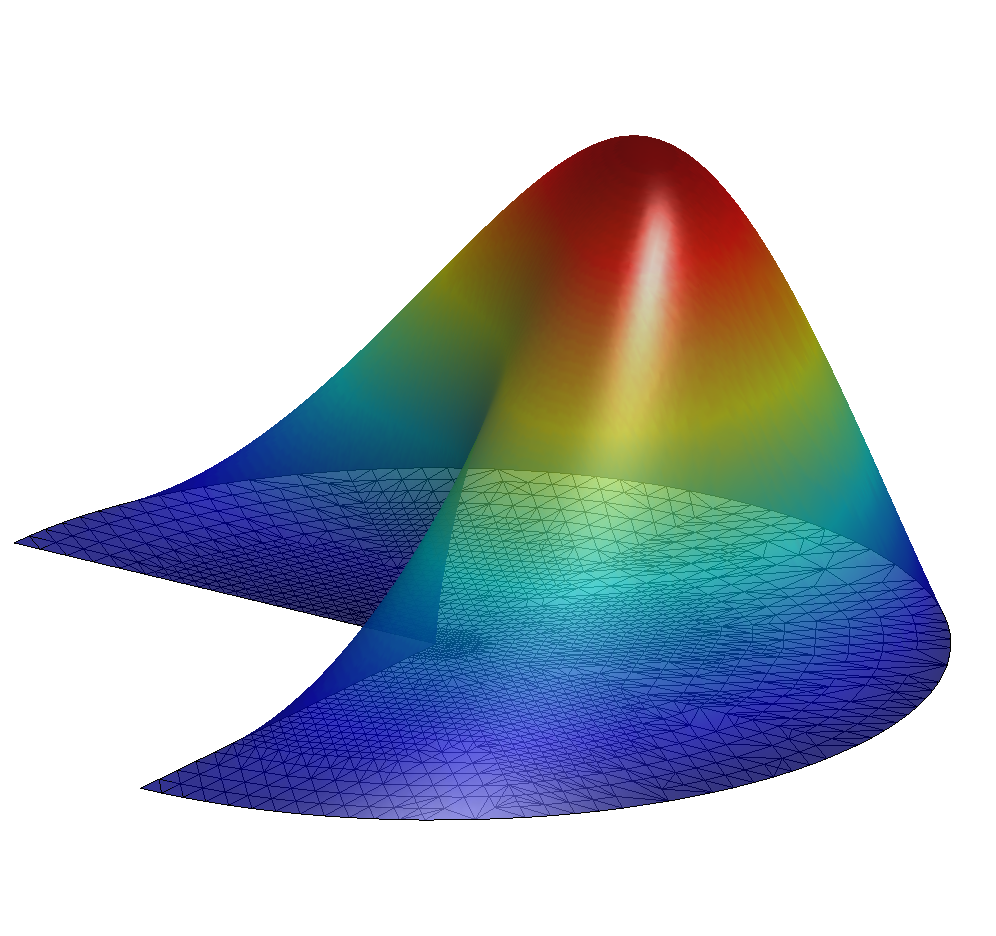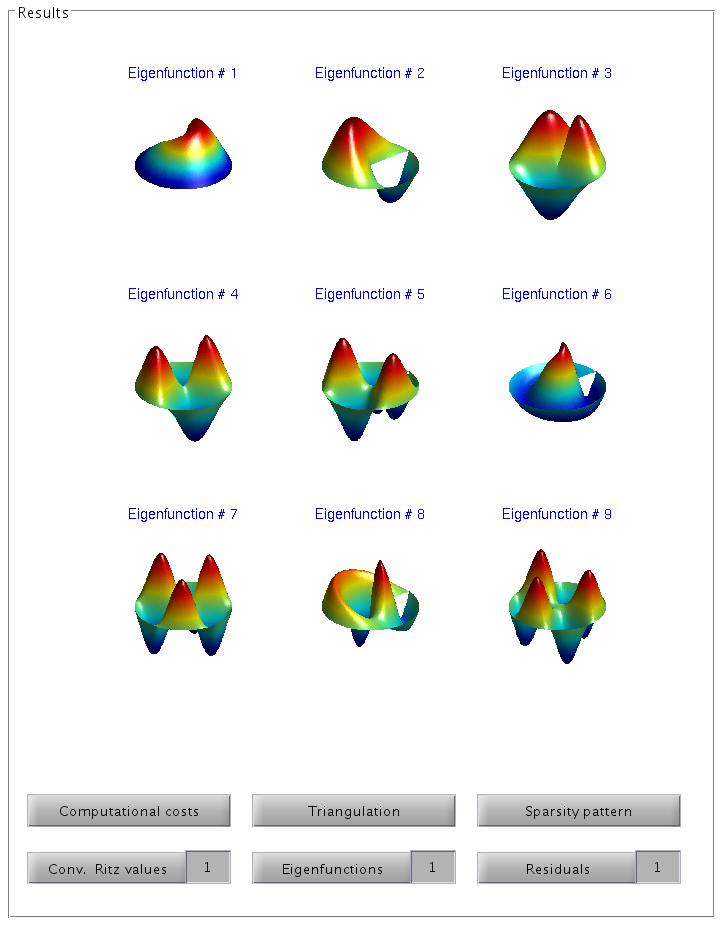# AMP Eigensolver

Version 1.0, July 2014.
Authors: Ming Zhou and Klaus Neymeyr at the Universität Rostock, Institut für Mathematik, Germany.

The AMP Eigensolver (Adaptive Multigrid Preconditioned Eigensolver) is a software for computing the smallest eigenvalues and the associated eigenfunctions of a self-adjoint and elliptic partial differential operator in 2D domains. The AMP Eigensolver software contains a fast adaptive grid refinement and an efficient iterative eigensolver with multigrid preconditioning. The adaptive grid refinement uses residual based error estimators with respect to linear and quadratic finite elements. The eigensolver is an implementation of the preconditioned gradient subspace iteration for the Rayleigh quotient. The preconditioner is a multigrid V-cycle with Jacobi smoothing. See  for numerical experiments with the AMP Eigensolver.
The core of the software is written in FORTRAN and uses the BLAS and LAPACK libraries. The FORTRAN code has been precompiled for the following platforms:

• Linux 32/64 bit,
• Mac OS 32/64 bit,
• Windows 32/64 bit.

The users' front-end is a graphical user interface (GUI) in Matlab.

• Software package: ampe.zip (7.2 MB ZIP file, optimized for Matlab 2013a) or ampe1.zip (with a slightly modified GUI for newer Matlab versions).
• Manual: ampe.pdf (1.7 MB PDF file).

### References:

1. Z. Bai, J. Demmel, J. Dongarra, A. Ruhe, and H. van der Vorst, editors. Templates for the solution of algebraic eigenvalue problems: A practical guide. SIAM, Philadelphia, 2000.
2. E. G. D'yakonov. Optimization in solving elliptic problems. CRC Press, Boca Raton, Florida, 1996.
3. W. Hackbusch. On the computation of approximate eigenvalues and eigenfunctions of elliptic operators by means of a multi-grid method. SIAM Journal on Numerical Analysis 16 (1979), 201-215.
4. A. V. Knyazev. Toward the Optimal Preconditioned Eigensolver: Locally Optimal Block Preconditioned Conjugate Gradient Method. SIAM Journal on Scientific Computing 23 (2001), 517-541.
5. A. V. Knyazev, M. E. Argentati, I. Lashuk and E. E. Ovtchinnikov. Block Locally Optimal Preconditioned Eigenvalue Xolvers (BLOPEX) in hypre and PETSc. SIAM Journal on Scientific Computing 29 (2007), 1267-1280.
6. A. V. Knyazev and K. Neymeyr. A geometric theory for preconditioned inverse iteration. III: A short and sharp convergence estimate for generalized eigenvalue problems. Linear Algebra Appl. 358 (2003), 95-114.
7. D. E. Longsine and S. F. McCormick. Simultaneous Rayleigh-quotient minimization methods for Ax = λBx. Linear Algebra Appl. 34 (1980), 195-234.
8. K. Neymeyr. A posteriori error estimation for elliptic eigenproblems. Numer. Linear Algebra Appl. 9 (2002), 263-279.
9. K. Neymeyr and M. Zhou. The block preconditioned steepest descent iteration for elliptic operator eigenvalue problems. Electron. Trans. Numer. Anal. 41 (2014), 93-108.

### Two screenshots of the AMP Eigensolver: